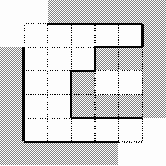## Ural 1033 Labyrinth

 Accepted 0.015 217 KB

## 1033. Labyrinth

Time Limit: 1.0 second
Memory Limit: 16 MB
Administration of the labyrinth has decided to start a new season with new wallpapers. For this purpose they need a program to calculate the square of the walls inside the labyrinth. This job is just for you!
The labyrinth is represented by a matrix N×N (3 ≤ N ≤ 33, you see, ‘3’ is a magic digit!). Some matrix cells contain a dot character (‘.’) that denotes an empty square. Other cells contain a diesis character (‘#’) that denotes a square filled by monolith block of stone wall. All squares are of the same size 3×3 meters.
The walls are constructed around the labyrinth (except for the upper left and lower right corners, which are used as entrances) and on the cells with a diesis character. No other walls are constructed. There always will be a dot character at the upper left and lower right corner cells of the input matrix.Your task is to calculate the square of visible part of the walls inside the labyrinth. In other words, the square of the walls' surface visible to a visitor of the labyrinth. Note that there's no holes to look or to move through between any two adjacent blocks of the wall. The blocks are considered to be adjacent if they touch each other in any corner. See picture for an example: visible walls inside the labyrinth are drawn with bold lines. The height of all the walls is 3 meters.

### Input

The first line of the input contains the single number N. The next N lines contain N characters each. Each line describes one row of the labyrinth matrix. In each line only dot and diesis characters will be used and each line will be finished with a new line character. There will be no spaces in the input.

### Output

Your program should print to the output a single integer — the exact value of the square of the wallpaper needed.

### Sample

input output
```5
.....
...##
..#..
..###
.....
```
```198
```

wa了两次 找不出错误，search(i+1，j)写成search(i+1,j+1)；
#include<iostream>
#include
<cstring>
using namespace std;

int const maxSize=35;

class ural1033
{
public:
ural1033(){ size
=0; memset(f,0,sizeof f); }

void input();

void print();

void search(int i, int j);

int size;

int getn(){return N;}
private:

char a[maxSize][maxSize];

bool f[maxSize][maxSize];

int N;
};

void ural1033::input()
{
cin
>>N;

int i,j;

for(i=1; i<=N; i++)

for(j=1; j<=N; j++)
cin
>>a[i][j];

for(i=2; i<=N+1; i++)a[i]='#';

for(i=2; i<=N+1; i++)a[i]='#';

for(i=1; i<=N-1; i++)a[N+1][i]='#';

for(i=1; i<=N-1; i++)a[i][N+1]='#';

}

void ural1033:: search(int i, int j)
{

if(i<1||i>N||j<1||j>N||a[i][j]=='#'||f[i][j]==1)return ;
f[i][j]
=1;

if(a[i-1][j]=='#')size++;

else search(i-1,j);

if(a[i][j-1]=='#')size++;

else search(i,j-1);

if(a[i][j+1]=='#')size++;

else search(i,j+1);

if(a[i+1][j]=='#')size++;

else search(i+1,j);
}

void ural1033::print()
{

for(int i=0; i<=N+1; i++,cout<<endl)

for(int j=0; j<=N+1; j++)
cout
<<a[i][j]<<' ';
cout
<<endl<<endl;

for(int i=0; i<=N+1; i++,cout<<endl)

for(int j=0; j<=N+1; j++)
cout
<<f[i][j]<<' ';
}

int main()
{
ural1033 ural;
ural.input();

ural.search(
1,1);
ural.search(ural.getn(),ural.getn());

cout
<<ural.size*9<<endl;

system(
"pause");

return 0;
}

posted on 2010-06-24 23:02 田兵 阅读(264) 评论(0)  编辑 收藏 引用 所属分类: URAL< 2010年6月 >
303112345
6789101112
13141516171819
20212223242526
27282930123
45678910

• 随笔 - 65
• 文章 - 2
• 评论 - 17
• 引用 - 0

•

• 积分 - 24167
• 排名 - 662